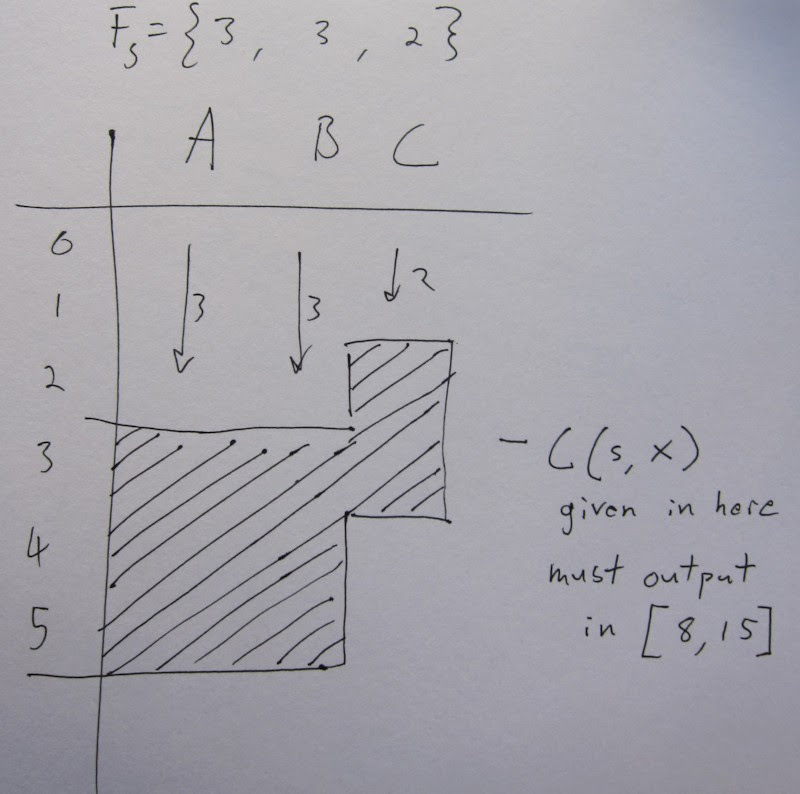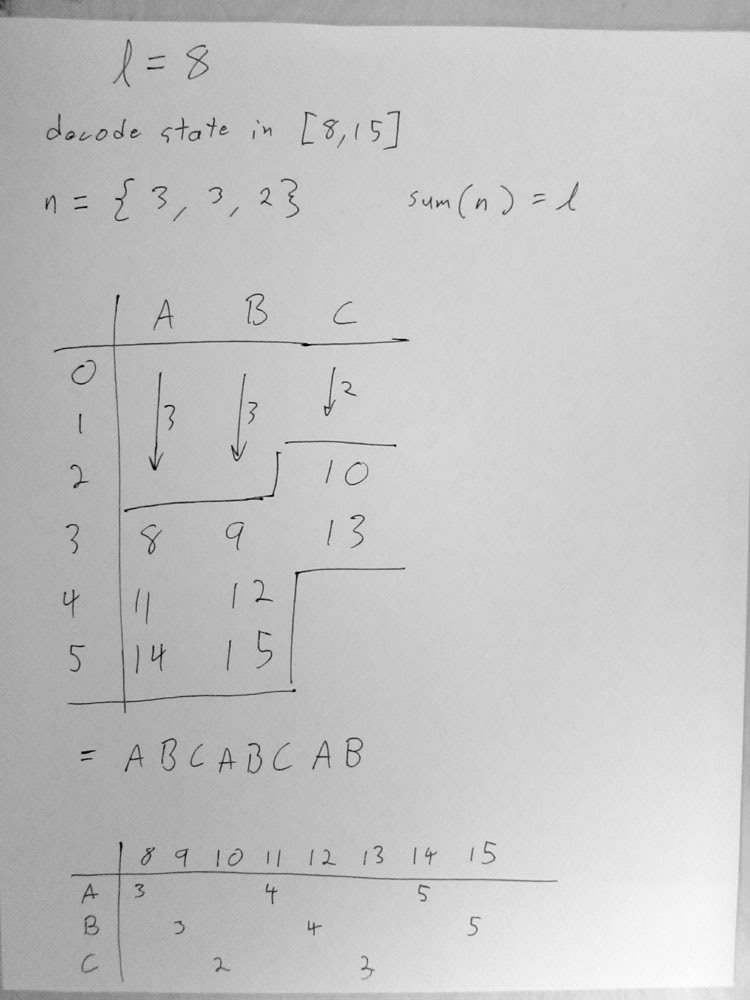cbloom rants

2/05/2014

02-05-14 - Understanding ANS - 7

And we're ready to cover table-based ANS (or "tANS") now.

I'm going to be quite concrete and consider a specific choice of implementation, rather than leaving everything variable. But extrapolation to the general solution is straightforward.

You have integer symbol frequences Fs. They sum to M. The cumulative frequencies are Bs.

I will stream the state x in bits. I will use the smallest possible renormalization range for this example , I = [ M , 2*M - 1]. You can always use any integer multiple of M that you want (k*M, any k), which will give you more coding resolution (closer to entropy). This is equivalent to scaling up all the F's by a constant factor, so it doesn't change the construction here.

Okay. We will encode/decode symbols using this procedure :

ENCODE                      DECODE

|                           ^
V                           |

stream out                  stream in

|                           ^
V                           |

C(s,x) coding function      D(x) decoding function

|                           ^
V                           |

x'                          x'

We need tables for C() and D(). The constraints are :

D(x') = { x , s }  outputs a state and a symbol

D(x) must be given for x in I = [ M , 2*M - 1 ]

D(x) in I must output each symbol s Fs times

that is, D(x in I) must be an output string made from a permutation of "AA..BB.." , each symbol Fs times

D( C( s, x ) ) = { x , s }  decode must invert coding

C(s,x) = x'  outputs the following state

C(s,x) must be given for x' in I
that's x in Is

The precursor ranges Is = { x : C(s,x) is in I }
must exist and be of the form Is = [ k , 2k-1 ] for some k

Now, if we combine the precursor range requirement and the invertability we can see :

D(x in I) outputs each s Fs times

C(s,x) with x' in I must input each s Fs times

the size of Is must be Fs

the precursor ranges must be Is = [ Fs, 2*Fs - 1 ]

C(s,x) must given in M slots

And I believe that's it; those are the necessary and sufficient conditions to make a valid tANS system. I'll go over some more points and fill in some details.

Here's an example of the constraint for an alphabet of "ABC" and M = 8 :Now, what do you put in the shaded area? You just fill in the output states from 8-15. The order you fill them in corresponds to the output string. In this case the output string must be some permutation of "AAABBBCC".

Here's one way : (and in true Duda style I have confusingly used different notation in the image, since I drew this a long time ago before I started this blog series. yay!)In the image above I have also given the corresponding output string and the decode table. If you're following along in Duda's paper arxiv 1311.2540v2 this is figure 9 on page 18. What you see in figure 9 is a decode table. The "step" part of figure 9 is showing one method of making the sort string. The shaded bars on the right are showing various permutations of an output string, with a shading for each symbol.

Before I understood ANS I was trying tables like this :

M=16
Fs = {7,6,3}

S |  0|  1|  2
---|---|---|---
1|  2|  3|  4
2|  5|  6| 10
3|  7|  8| 15
4|  9| 11| 20
5| 12| 13| 26
6| 14| 16| 31
7| 17| 19|
8| 18| 22|
9| 21| 24|
10| 23| 27|
11| 25| 29|
12| 28|   |
13| 30|   |

This table does not work. If you're in state x = 7 and you want to encode symbol 2, you need to stream out bits to get into the precursor range I2. So you stream out from x=7 and get to x=3. Now you look in the table and you are going to state 15 - that's not in the range I=[16,31]. No good!

A correct table for those frequencies is :

S |  0|  1|  2
---|---|---|---
3|   |   | 18
4|   |   | 24
5|   |   | 29
6|   | 17|
7| 16| 20|
8| 19| 22|
9| 21| 25|
10| 23| 27|
11| 26| 31|
12| 28|   |
13| 30|   |

Building the decode table from the encode table is trivial.

Note that the decode table D(x) only has to be defined for x in I - that's M entries.

C(x,s) also only has M entries. If you made it naively as a 2d array, it would be |alphabet|*M . eg. something like (256*4096) slots, but most of it would be empty. Of course you don't want to do that.

The key observation is that C(x,s) is only defined over consecutive ranges of x for each s. In fact it's defined over [Fs, 2*Fs-1]. So, we can just pack these ranges together. The starting point in the packed array is just Bs - the cumulative frequency of each symbol. That is :

PC = packed coding table
PC has M entries

C(x,s) = PC[ Bs + (x - Fs) ]

eg. for the {3,3,2} table shown in the image above :

PC = { 8,11,14, 9,12,15, 10,13 }

this allows you to store the coding table also in an array of size M.

There are a few topics on tANS left to cover but I'll leave them for the next post.